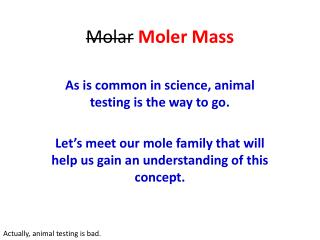DownloadDownload PresentationMolar Moler Mass

# Molar Moler Mass

Télécharger la présentation## Molar Moler Mass

- - - - - - - - - - - - - - - - - - - - - - - - - - - E N D - - - - - - - - - - - - - - - - - - - - - - - - - - -
##### Presentation Transcript

1. MolarMoler Mass As is common in science, animal testing is the way to go. Let’s meet our mole family that will help us gain an understanding of this concept. Actually, animal testing is bad.

2. But first, what is Molar Mass? • The molar mass is the sum of the atomic masses of every atom of every element present in a compound. • This concept is crucial in industry as companies can use this information (as well as Unit 7) to calculate how much a reaction will cost and how much product they can sell to make profit. • This means the calculation will involve addition (which I know you can do) and multiplication (which I also know you can do). • As we learned in Unit 4, we can find the atomic mass on the periodic table.

3. The Mole Family - Dad • Dad, symbol – D, is fat and quite happy. He measures in with a mass of 375.5 g.

4. The Mole Family - Mom • Mom, symbol - M, is relaxing after taking care of the kids all day. She is much smaller than her husband, with a mass of 235.75 g.

5. The Mole Family - Babies • The babies, symbol – B, each come in with a mass of 4.25 g.

6. The Mole Family - Mass • What is the mass of this family with a formula of DMB3?

7. The Mole Family - Mass • Find the mass associated with each individual • D (Dad) = 375.5 g • M (Mom) = 235.75 g • B3 (Babies) = 4.25 g x 3 = 12.75 g • Now add your answers together • 375.5 + 235.75 + 12.75 = 624.00 g • The moler mass is 624.00 g. • Piece of cake, right?